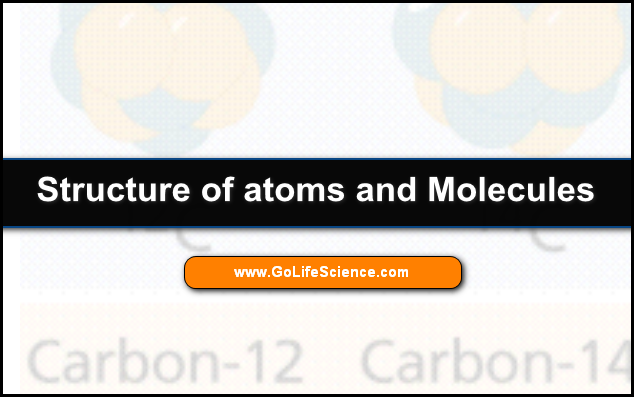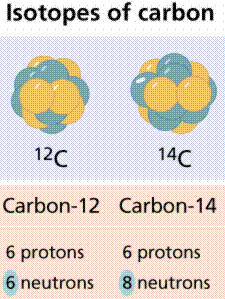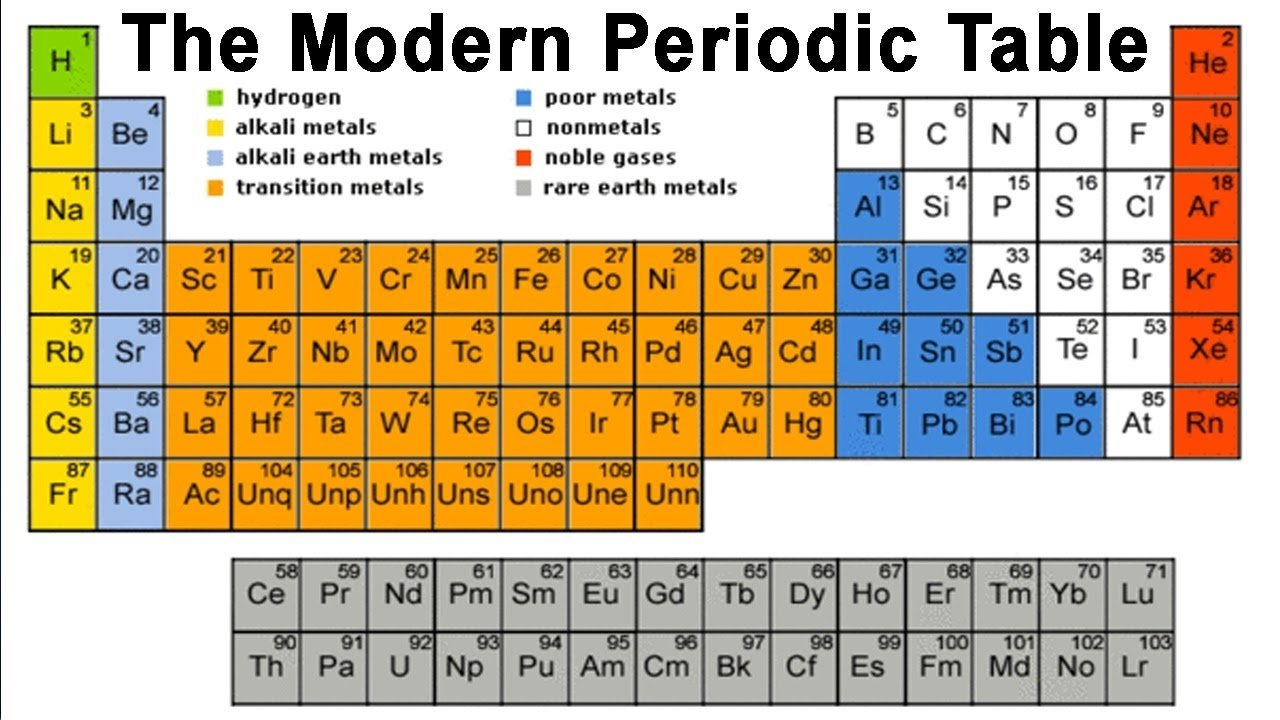# Structure of Atoms and Molecules (Basic Chemistry)

Most of the Universe consists of matter and energy. Energy is the capacity to do work. The matter has mass and occupies space. All matter is composed of basic elements that cannot be broken down into substances with different chemical or physical properties. Elements are substances consisting of one type of atom, for example, Carbon atoms make up the diamond, and also graphite. Pure (24K) gold is composed of only one type of atom, gold atoms. Atoms are the smallest particle into which an element can be divided. Now let us go in detail of Structure of Atoms and Molecules.## Structure of Atoms and Molecules:

The ancient Greek philosophers developed the concept of the atom, although they considered it the fundamental particle that could not be broken down. Since the work of Enrico Fermi and his colleagues, we now know that the atom is divisible, often releasing tremendous energies as in nuclear explosions or (in a controlled fashion in) thermonuclear power plants. This structure of atoms and molecules unit explains the basic part of atoms and molecules.

Subatomic particles were discovered during the 1800s. For our purposes, we will concentrate only on three of them, summarized in Table 1. The proton is located at the canter (or nucleus) of an atom, each atom has at least one proton.

• Protons have a charge of +1, and a mass of approximately 1 atomic mass unit (AMU). Elements differ from each other in the number of protons they have, e.g. Hydrogen has 1 proton; Helium has 2.
• The neutron also is located in the atomic nucleus (except in Hydrogen). The neutron has no charge and a mass of slightly over 1 amu. Some scientists propose the neutron is made up of a proton and electron-like particle.
• The electron is a very small particle located outside the nucleus. Because they move at speeds near the speed of light the precise location of electrons is hard to pin down. Electrons occupy orbitals or areas where they have a high statistical probability of occurring. The charge on an electron is -1. Its mass is negligible (approximately 1800 electrons are needed to equal the mass of one proton).

Table 1. Subatomic particles of use in biology.

 Name Charge Location Mass Proton +1 atomic nucleus 1.6726 X 10-27 kg Neutron 0 atomic nucleus 1.6750 X 10-27 kg Electron -1 electron orbital 9.1095 X 10-31 kg
• The atomic number is the number of protons an atom has. It is characteristic and unique for each element.
• The atomic mass (also referred to as the atomic weight) is the number of protons and neutrons in an atom.
• Atoms of an element that have differing numbers of neutrons (but a constant atomic number) are termed isotopes.
Biochemical pathways can be deciphered by using isotopic tracers. The age of fossils and artifacts can be determined by using radioactive isotopes, either directly on the fossil (if it is young enough) or on the rocks that surround the fossil (for older fossils like dinosaurs). Isotopes are also the source of radiation used in medical diagnostic and treatment procedures.Figure 1. Note that each of these isotopes of hydrogen has only one proton. Isotopes differ from each other in the number of neutrons, not in the number of protons.
Some isotopes are radioisotopes, which spontaneously decay, releasing radioactivity. Other isotopes are stable. Examples of radioisotopes are Carbon-14 (symbol 14C), and deuterium (also known as Hydrogen-2; 2H). Stable isotopes are 12C and 1H.Figure 2. Carbon has three isotopes, of which carbon-12 and carbon-14 are the most well known.
The Periodic Table of the Elements, a version of which is shown in Figure 3, provides a great deal of information about various elements. Check the Elements periodic table now.Figure 3. The Periodic Table of the Elements. Each Roman numerical column on the label (at least the ones ending in A) tells us how many electrons are in the outer shell of the atom. Each numbered row on the table tells us how many electron shells an atom has. Thus, Hydrogen, in column IA, row 1 has one electron in one shell. Phosphorous in column VA, row 3 has 5 electrons in its outer shell and has three shells in total. Image from James K. Hardy’s chemistry site at the University of Akron.

## Electrons and energy:

Electrons, because they move so fast (approximately at the speed of light), seem to straddle the fence separating energy from matter. Albert Einstein developed his famous E=mc2 equation relating matter and energy over a century ago. Because of his (and others) work, we think of electrons both as particles of matter (having mass is a property of matter) and as units (or quanta) of energy. When subjected to energy, electrons will acquire some of that energy.

An orbital is also an area of space in which an electron will be found 90% of the time. Orbitals have a variety of shapes. Each orbital has a characteristic energy state and a characteristic shape.

The s orbital is spherical. Since each orbital can hold a maximum of two electrons, atomic numbers above 2 must fill the other orbitals. The px, py, and pz orbitals are dumbbell-shaped, along with the x, y, and z-axis respectively. These orbital shapes are shown in Figure 5.

Energy levels (also referred to as electron shells) are located a certain “distance” from the nucleus. The major energy levels into which electrons fit, are (from the nucleus outward) K, L, M, and N.

Sometimes these are numbered, with electron configurations being: 1s22s22p1, (where the first shell K is indicated with the number 1, the second shell L with the number 2, etc.). This nomenclature tells us that for the atom mentioned in this paragraph, the first energy level (shell) has two electrons in its s orbital (the only orbital it can have), and second energy level has a maximum of two electrons in its s orbital, plus one electron in its p orbital.